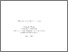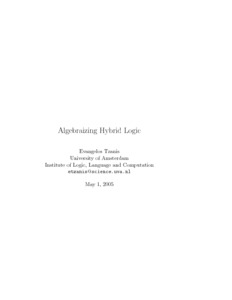# MoL-2005-03: Algebraizing Hybrid Logic

MoL-2005-03: Tzanis, Evangelos (2005) Algebraizing Hybrid Logic. [Report]Preview Text (Full Text) MoL-2005-03.text.pdf Download (303kB) | PreviewText (Abstract) MoL-2005-03.abstract.txt Download (1kB)

## Abstract

Algebraizing Hybrid Logic
Evangelos Tzanis

Abstract:
Hybrid logic is the result of extending the basic modal language with
a second sort of atomic propositions called nominals, and with
satisfaction operators. Precisely, the nominals (denoted by i, j, ...)
behave similar to ordinary proposition letters, expect that nominals
are true uniquely at a world. In other words, a nominal names a state
by being true there and nowhere else. An example of a formula
involving nominals is \diamond\diamond i \implies \neg\diamond i. The
language obtained by adding nominals to the basic modal language, is
called the minimal hybrid logic H. Satisfaction operators allow one to
express that a formula holds at the world named by nominal. A formula
of the form @_i\varphi expresses that p holds at the world named by
the nominal i. The extension of the basic modal language with nominals
and satisfaction operators is called the basic hybrid language H(@).

In this thesis we introduce and study an extension of hybrid logic in
which the set of nominals may be endowed with an algebraic
structure. In other words we add modal operators only for
nominals. The main motivation of the paper comes from : you can
name states but you can not give them structure. In this paper we
consider an application of hybrid logics to relational structures on
algebras, thus a set with a relation and an algebraic
structure. Roughly speaking we try to give to nominals a structure, we
study the case where this structure is an algebraic structure. As far
as we know, the possible algebraic structure of nominals has not been
studied in the context of hybrid logic before. We should note that in
 there is a complete list of papers which study Kripke frames in
which the universe of possible worlds has a specific algebraic
structure, besides the traditional relational component.

Item Type: Report MoL-2005-03 Master of Logic Thesis (MoL) Series 2005 hybrid logic, algebraization Logic 12 Oct 2016 14:38 12 Oct 2016 14:38 https://eprints.illc.uva.nl/id/eprint/757View Item